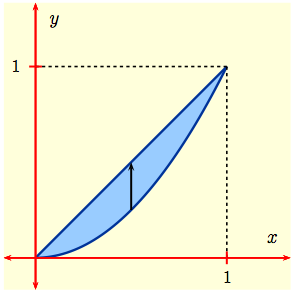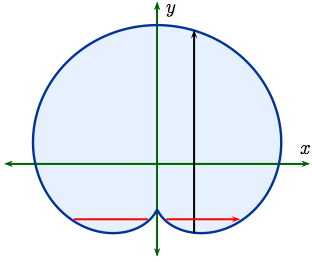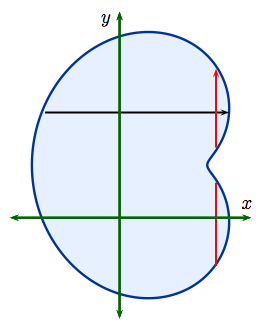Home

#### Integration by Parts

Integration by Parts
Examples
Integration by Parts with a definite integral
Going in Circles

#### Integrals of Trig Functions

Antiderivatives of Basic Trigonometric Functions
Product of Sines and Cosines (mixed even and odd powers or only odd powers)
Product of Sines and Cosines (only even powers)
Product of Secants and Tangents
Other Cases

#### Trig Substitutions

How Trig Substitution Works
Summary of trig substitution options
Examples
Completing the Square

#### Partial Fractions

Introduction to Partial Fractions
Linear Factors
Improper Rational Functions and Long Division
Summary

#### Strategies of Integration

Substitution
Integration by Parts
Trig Integrals
Trig Substitutions
Partial Fractions

#### Improper Integrals

Type 1 - Improper Integrals with Infinite Intervals of Integration
Type 2 - Improper Integrals with Discontinuous Integrands
Comparison Tests for Convergence

#### Modeling with Differential Equations

Introduction
Separable Equations
A Second Order Problem

#### Euler's Method and Direction Fields

Direction Fields
Euler's method revisited

#### Separable Equations

The Simplest Differential Equations
Separable differential equations
Mixing and Dilution

#### Models of Growth

Exponential Growth and Decay
The Zombie Apocalypse (Logistic Growth)

#### Linear Equations

Linear ODEs: Working an Example
The Solution in General
Saving for Retirement

#### Parametrized Curves

Three kinds of functions, three kinds of curves
The Cycloid
Visualizing Parametrized Curves
Tracing Circles and Ellipses
Lissajous Figures

#### Calculus with Parametrized Curves

Video: Slope and Area
Video: Arclength and Surface Area
Summary and Simplifications
Higher Derivatives

#### Polar Coordinates

Definitions of Polar Coordinates
Graphing polar functions
Video: Computing Slopes of Tangent Lines

#### Areas and Lengths of Polar Curves

Area Inside a Polar Curve
Area Between Polar Curves
Arc Length of Polar Curves

#### Conic sections

Slicing a Cone
Ellipses
Hyperbolas
Parabolas and Directrices
Shifting the Center by Completing the Square

#### Conic Sections in Polar Coordinates

Foci and Directrices
Visualizing Eccentricity
Astronomy and Equations in Polar Coordinates

#### Infinite Sequences

Examples of Infinite Sequences
Limit Laws for Sequences
Theorems for and Examples of Computing Limits of Sequences
Monotonic Covergence

#### Infinite Series

Introduction
Geometric Series
Limit Laws for Series
Test for Divergence and Other Theorems
Telescoping Sums

#### Integral Test

Preview of Coming Attractions
The Integral Test
Estimates for the Value of the Series

#### Comparison Tests

The Basic Comparison Test
The Limit Comparison Test

#### Convergence of Series with Negative Terms

Introduction, Alternating Series,and the AS Test
Absolute Convergence
Rearrangements

The Ratio Test
The Root Test
Examples

#### Strategies for testing Series

Strategy to Test Series and a Review of Tests
Examples, Part 1
Examples, Part 2

#### Power Series

Finding the Interval of Convergence
Power Series Centered at $x=a$

#### Representing Functions as Power Series

Functions as Power Series
Derivatives and Integrals of Power Series
Applications and Examples

#### Taylor and Maclaurin Series

The Formula for Taylor Series
Taylor Series for Common Functions
Adding, Multiplying, and Dividing Power Series
Miscellaneous Useful Facts

#### Applications of Taylor Polynomials

Taylor Polynomials
When Functions Are Equal to Their Taylor Series
When a Function Does Not Equal Its Taylor Series
Other Uses of Taylor Polynomials

#### Functions of 2 and 3 variables

Functions of several variables
Limits and continuity

#### Partial Derivatives

One variable at a time (yet again)
Definitions and Examples
An Example from DNA
Geometry of partial derivatives
Higher Derivatives
Differentials and Taylor Expansions

#### Differentiability and the Chain Rule

Differentiability
The First Case of the Chain Rule
Chain Rule, General Case
Video: Worked problems

#### Multiple Integrals

General Setup and Review of 1D Integrals
What is a Double Integral?
Volumes as Double Integrals

#### Iterated Integrals over Rectangles

How To Compute Iterated Integrals
Examples of Iterated Integrals
Fubini's Theorem
Summary and an Important Example

#### Double Integrals over General Regions

Type I and Type II regions
Examples 1-4
Examples 5-7
Swapping the Order of Integration
Area and Volume Revisited

#### Double integrals in polar coordinates

dA = r dr (d theta)
Examples

#### Multiple integrals in physics

Double integrals in physics
Triple integrals in physics

#### Integrals in Probability and Statistics

Single integrals in probability
Double integrals in probability

#### Change of Variables

Review: Change of variables in 1 dimension
Mappings in 2 dimensions
Jacobians
Examples
Bonus: Cylindrical and spherical coordinates

### Examples 5-7

In the previous Examples 3 and 4, the way we specified $D$ and $R$ suggested how to write the integral as a iterated integral.  Sometimes conditions are best interpreted graphically before deciding on whether to evaluate as Type I or Type II.

Example 5:  Evaluate the integral $\displaystyle I \ = \iint_D\, (3x +4y)\, dA$,
where $D$ is the bounded region enclosed by $y = x$ and $y=x^2$.

DO:  Without looking, graph the region $D$.  Set up a Type I integral.

 Solution 5:  $D$ is enclosed by the straight line $y = x$ and the parabola $y = x^2$ as shown here. To determine the limits of integration we first need to find the points of intersection of $y = x$ and $y = x^2$. These occur when $x^2 = x$.  This means $x(x-1)=0$, so $x = 0,\, 1$. Treating $D$ as a Type I region, we fix $x$ between $x=0$ and $x=1$, and integrate with respect to $y$ along the black vertical line, getting the iterated integral $\displaystyle I \ = \ \int_0^1\left(\int_{x^2}^{x}\, (3x + 4y)\, dy\right)\,dx$  DO:  Evaluate $I$.------------------------------------------------------------------------------

In Example 5 it was simpler to fix $x$ and integrate first with respect to $y$ because the bounding curves were already given to us in terms of $x$, as $$y \ = \ f_1(x)\ = \ x^2\,,\qquad y \ = \ f_2(x)\ = \ x\,.$$ Had they been given as $x \,=\, g_1(y)$ and $x\,=\, g_2(y)\,$ it might have been easier to think of $D$ as a Type II region. We would fix $y$ and first integrate with respect to $x$.  Sometimes the geometry of the region of integration makes it obvious whether we have Type I or Type II, as in the following examples (which we will not evaluate).

 Example 6:  The region $D$ that is shown here is Type I but is not Type II:  Fixing $x$ and integrating first with respect to $y$ along the vertical black line makes good sense because then we have the same curve $f_1(x)$ along the bottom and $f_2(x)$ along the top: $$D \ = \ \Bigl\{\,(x,\,y) : f_1(x) \le y \le f_2(x),\ \ a \le x \le b\,\Bigl\}$$ for suitable choices of $a,\, b$ and functions $f_1(x),\, f_2(x)$ giving us: $$\iint_D\, f(x,\,y)\, dA = \int_a^b \left(\int_{f_1(x)}^{f_2(x)}\, f(x,\,y)\, dy\right) dx\,.$$But if we had chosen to fix $y$, then the integral with respect to $x$ would sometimes split into two parts, as is shown with the red horizontal lines.  This would make evaluate of this integral more complicated -- for one thing, there would be more than one integral.------------------------------------------------------------------------------

 Example 7:  Similarly, the region $D$ shown here is Type II but not Type I.  Fixing $y$ and integrating first with respect to $x$ along the horizontal black line makes good sense because then $$D \ = \ \Bigl\{\,(x,\,y) : g_1(y) \le x \le g_2(y),\ \ c \le y \le d\,\Bigl\}$$ for suitable choices of $c,\, d$ and functions $g_1(y),\, g_2(y)$.  In this case $$\iint_D\, f(x,\,y)\, dxdy = \int_c^d \left(\int_{g_1(y)}^{g_2(y)}\, f(x,\,y)\, dx\right) dy\,.$$But if we had chosen to fix $x$, then the integral with respect to $y$ would sometimes splits into two parts as shown by the red vertical lines.  Again, this would make the integral(s) more complicated.